Circles

# Class 10 Math Chapter 10 Circles Important QuestionsHere are some important questions for Class 10 Mathematics Chapter 10, Circles, carefully selected to help students prepare for the CBSE Class 10 Mathematics Examination in 2023-24. These questions cover various types of problems and are designed to assist students in understanding Circles better. By practicing these diverse question types, students can clarify any doubts they may have and improve their problem-solving skills, leading to better performance in the chapter on Circles.

## Introduction

In Chapter 10 of Class 10 Mathematics, Circles, In this chapter, we explore the properties of tangents to a circle at the point of contact. Two important properties are:

1. (Prove) The tangent at any point of a circle is perpendicular to the radius through the point of contact.
2. (Prove) The lengths of tangents drawn from an external point to a circle are equal.

### Define a tangent to a circle

A tangent to a circle is a straight line that touches the circle at exactly one point, known as the point of tangency. This point of contact is where the tangent line intersects the circle's circumference. The tangent line is always perpendicular to the radius drawn to the point of tangency.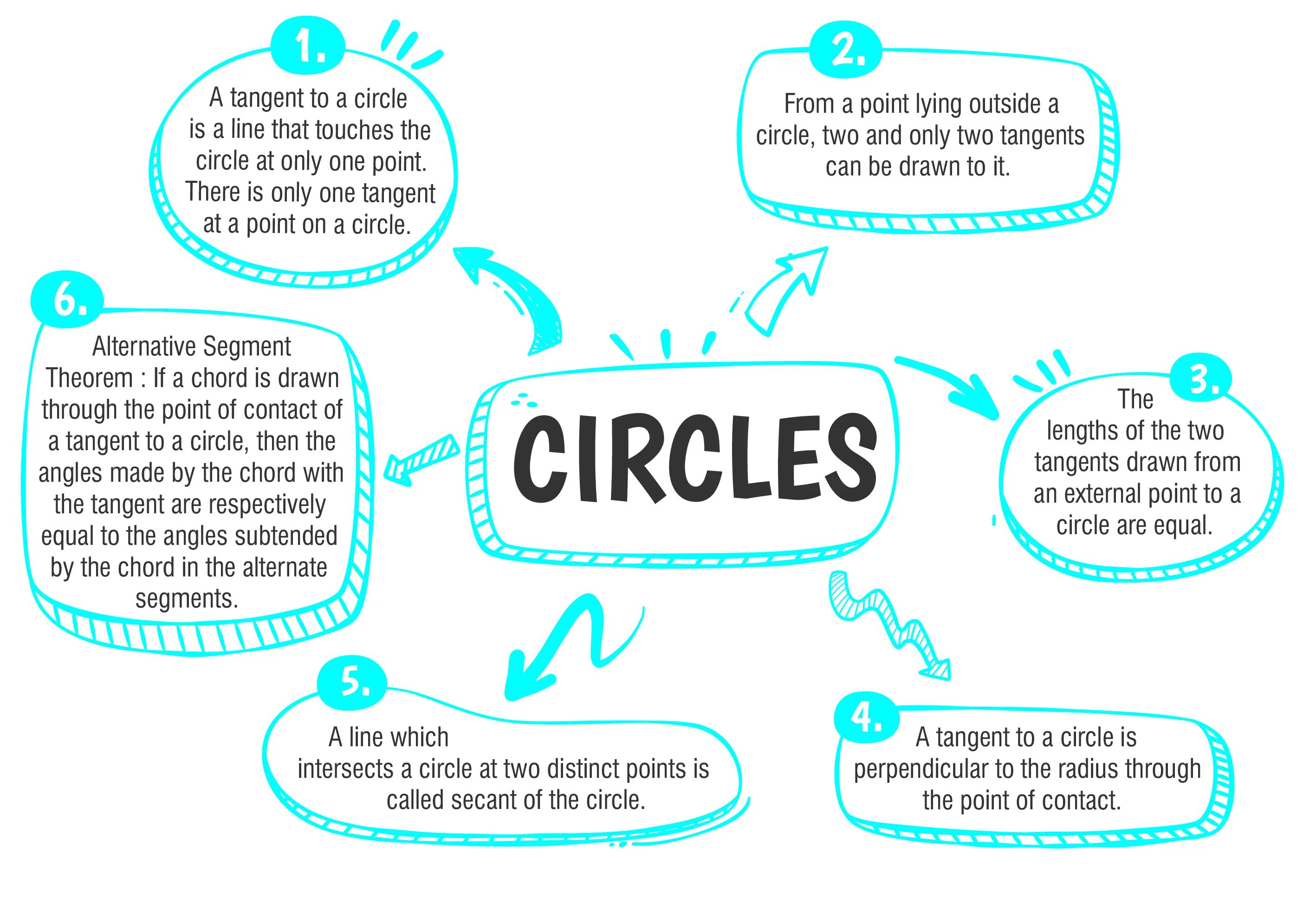### Class 10 Circles Important Questions and Answers

#### Options

Ans. (b) 9 cm

Explanation:
Since, PA and PB are tangents to the circle  from point P.
Therefore PA = PB …(i)
Also, CD is the tangent touching the circle at Q.
Therefore,  CA = CQ and DQ = DB …(ii)
Since, tangents from external points are equal.
Now, PA = PB = 12 cm
CA = CQ = 3 cm
PC = PA - CA
= 12 - 3 = 9 cm.

#### (a) 1 cm(b) 2 cm(c) 3 cm(d) 4 cm

Ans. (b) 2 cm

Explanation:
An incircle is drawn with centre O which touches the sides of the triangle ABC at P, Q and R. OP, OQ and OR are radii and AB, BC and CA are the tangents to the circle.
OP ⊥ AB, OQ ⊥ BC and OR ⊥ CA.
OPBQ is a square. ( ∵ ∠B - 90o)
Let r be the radius of the circle
PB = BQ = r
AR = AP = 8 - r,
CQ = CR = 6 - r
AC = AR + CR
⇒ 10 = 8 - r + 6 - r
⇒ 10 = 14 - 2r
⇒ 2r = 14 - 10 = 4
⇒ r = 2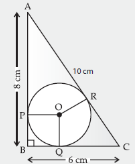#### Q3. The radii of two concentric circles are 13 cm and 8 cm. PQ is a diameter of the bigger circle. QR is a tangent to the smaller circle touching it at R. Find the length PR.

Ans. PR = 19 cm

Explanation:
Let the line QR intersect the bigger circle at S.
Join PS.
O is the mid-point of PQ. [∵ PQ is a diameter of the bigger circle]
QR is a tangent to the smaller circle and OR is a radius through the point of contact R.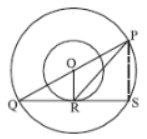∴ OR ⊥ QR ⇒ OR ⊥ QS
Since, OR is perpendicular to a chord QS of the bigger circle.
∴        QR = RS
[ ∵ Perpendicular from the centre to a chord bisects the chord]
$$\Rightarrow R is the mid-point of QS.$$
∴ In △QSP, O is the mid-point of PQ and R is the mid-point of QS.
$$\therefore$$ OR =$$\frac{1}{2}Ps$$
[∵ segment joining the mid-points of any two sides of a triangle is half of the third side]
⇒ PS = 2OR = 2 × 8 cm = 16 cm
In right △OQR, OR2 + QR2 = OQ2
⇒ 82 + QR2 = 132
⇒ 64 + QR2 = 169
⇒ QR2 = 169 – 64 = 105
QR =$$\sqrt{105}$$
$$\therefore$$ RS = QR =$$\sqrt{105}$$
In △PRS, PR2 = RS2 + PS
=$$\sqrt{105}^2+16^2$$
⇒ PR = 19 cm.

#### Q4. Shipra prepared a project for rain water harvesting. diagrammatic representation of the project is given in the figure. PQ and PR are the pipes touching the circular pit. Length of these pipes is 5 m each. What is the perimeter of ΔPMN?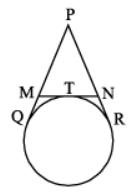Ans. Perimeter of △PMN = 10 cm.

Explanation:
Here, MT = MQ   [Tangents from point M]   ...(i)
And NT = NR [Tangents from point N] ...(ii)
Now,   PQ + PR = PM + MQ + PN + NR
= PM + MT + PN + NT [Using eq. (i) and (ii)]
= PM + PN + (MT + NT)
= PM + PN + MN      = Perimeter of DPMN
∴ Perimeter of △PMN      = 5 + 5
= 10 cm.

#### Q5. From an external point P, tangents PA and PB are drawn to a circle with centre O. If CD is the tangent to the circle at a point E and PA = 14 cm, find the perimeter of △PCD.

Ans. Perimeter of △PCD =  28 cm

Explanation:
Given, PA and PB are the tangents drawn from a point P outside the circle with centre O.CD is another tangents to the circle at point E which intersects PA and PB at C and D respectively.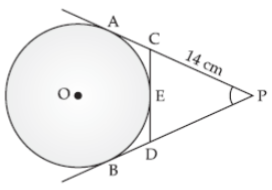Here, PA = 14 cm
PA and PB are the tangents to the circle from P
So, PA = PB = 14 cm
Now, CA and CE are the tangents from C to the circle.
∴ CA = CE …(i)
Now, perimeter of △PCD
= PC + PD + CD
= PC + PD + CE + DE
= PC + CE + PD + DE
= PC + CA + PD + DB
{From (i) and (ii)}
= PA + PB
= 14 + 14
= 28 cm#### CBSE Class 10 Maths Chapter wise Important Questions

Chapter No. Chapter Name
Chapter 1 Real Number
Chapter 2 Polynomials
Chapter 3 Pair of Linear Equations in Two Variables
Chapter 5 Arithmetic Progressions
Chapter 6 Triangles
Chapter 7 Coordinate Geometry
Chapter 8 Introduction to Trigonometry
Chapter 9 Some Applications of Trigonometry
Chapter 10 Circles
Chapter 11 Areas Related to Circle
Chapter 12 Surface Areas and Volumes
Chapter 13 Statistics
Chapter 14 Probability

#### Conclusion

If you want to get better at understanding the Circles chapter, you should check out oswal.io. This website offers lots of practice questions made to help you learn more effectively. By practicing these questions, you can improve how well you understand circles and get better at solving problems related to them. It's a really helpful way to practice and feel more confident when dealing with circle concepts.

#### Q1 : How is the diameter of a circle related to its radius?

Ans: The diameter of a circle is twice the length of its radius. In other words, diameter = 2 × radius.

#### Q2: What is the relationship between the radius, diameter, and circumference of a circle?What is the relationship between the radius, diameter, and circumference of a circle?

Ans: The circumference of a circle (C) is given by the formula C = 2πr, where 'r' is the radius. Also, the diameter (d) is twice the radius, so d = 2r.

#### Q3 : What is the angle between a tangent and a radius drawn to the point of tangency? The

Ans: angle between a tangent and a radius drawn to the point of tangency is 90 degrees, making them perpendicular to each other

#### Q4 : How is the length of a tangent segment related to the radius of the circle?

Ans: In a circle, tangents drawn from an external point are equal in length. They are both perpendicular to the radius drawn to the point of contact on the circle.

#### Q5 : What is the angle sum property of a quadrilateral formed by four tangents to a circle?

Ans: The angle sum of a quadrilateral formed by four tangents drawn to a circle is 360 degrees.

###### Copyright 2022 OSWAL PUBLISHERS Simplifying ExamsPhone:  (+91) 78959 87722
Mail: support@oswalpublishers.in